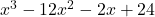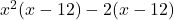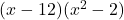## Which shows one way to determine the factors of x3 – 12×2 – 2x + 24 by grouping?

Question

Which shows one way
to determine the factors of x3 – 12×2 – 2x + 24 by grouping?

in progress 0
6 months 2021-07-21T20:31:00+00:00 2 Answers 7 views 0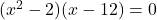Step-by-step explanation:

Factoring by grouping is a way of determining the factors of a polynomial equation. One firsts groups terms by putting them in parenthesis. Then one will take a common factor out of the parenthesis. Finally, one will group the coefficients and the grouped terms respectively, thus rewriting the polynomial equation as the product of its factors.

One is given the following equation: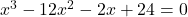Group the different terms in the equation: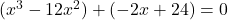Factor, write a common factor that both terms in the parenthesis share, outside of the parenthesis.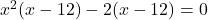Factor the equation again. Group the like terms together, and the coefficients together.